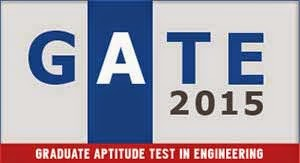# GATE 2015 syllabus, GATE 2015 syllabus for ME, Gate Syllabus for Mechanical Engineering, syllabus for gate 2015 mechanical engineering, gate 2015 syllabus for civil engineering, GATE 2015 Mechanical Engineering, GATE 2015 Syllabus, GATE Syllabus, gate syllabus for mechanical engineering 2015GATE 2015 Syllabus for Mechanical Engineering

## GATE 2015 Syllabus for Mechanical Engineering (ME)

### ENGINEERING MATHEMATICS

Linear Algebra: Matrix algebra, Systems of linear equations, Eigen values and eigen vectors.

Calculus: Functions of single variable, Limit, continuity and differentiability, Mean value
theorems, Evaluation of definite and improper integrals, Partial derivatives, Total derivative,
Maxima and minima, Gradient, Divergence and Curl, Vector identities, Directional derivatives,
Line, Surface and Volume integrals, Stokes, Gauss and Green’s theorems.

Differential equations: First order equations (linear and nonlinear), Higher order linear
differential equations with constant coefficients, Cauchy’s and Euler’s equations, Initial and
boundary value problems, Laplace transforms, Solutions of one dimensional heat and wave
equations and Laplace equation.

Complex variables: Analytic functions, Cauchy’s integral theorem, Taylor and Laurent series.
Probability and Statistics: Definitions of probability and sampling theorems, Conditional
probability, Mean, median, mode and standard deviation, Random variables, Poisson,Normal and
Binomial distributions.

Numerical Methods: Numerical solutions of linear and non-linear algebraic equations
Integration by trapezoidal and Simpson’s rule, single and multi-step methods for differential
equations.

### APPLIED MECHANICS AND DESIGN

Engineering Mechanics: Free body diagrams and equilibrium; trusses and frames; virtual work;
kinematics and dynamics of particles and of rigid bodies in plane motion, including impulse and
momentum (linear and angular) and energy formulations; impact.

Strength of Materials: Stress and strain, stress-strain relationship and elastic constants, Mohr’s
circle for plane stress and plane strain, thin cylinders; shear force and bending moment diagrams;
bending and shear stresses; deflection of beams; torsion of circular shafts; Euler’s theory of
columns; strain energy methods; thermal stresses.

Theory of Machines: Displacement, velocity and acceleration analysis of plane mechanisms;
dynamic analysis of slider-crank mechanism; gear trains; flywheels.

Vibrations: Free and forced vibration of single degree of freedom systems; effect of damping;
vibration isolation; resonance, critical speeds of shafts.

Design: Design for static and dynamic loading; failure theories; fatigue strength and the S-N
diagram; principles of the design of machine elements such as bolted, riveted and welded joints,
shafts, spur gears, rolling and sliding contact bearings, brakes and clutches.

### FLUID MECHANICS AND THERMAL SCIENCES

Fluid Mechanics: Fluid properties; fluid statics, manometry, buoyancy; control-volume analysis
of mass, momentum and energy; fluid acceleration; differential equations of continuity and
momentum; Bernoulli’s equation; viscous flow of incompressible fluids; boundary layer;
elementary turbulent flow; flow through pipes, head losses in pipes, bends etc.

Heat-Transfer: Modes of heat transfer; one dimensional heat conduction, resistance concept,
electrical analogy, unsteady heat conduction, fins; dimensionless parameters in free and forced
convective heat transfer, various correlations for heat transfer in flow over flat plates and through
pipes; thermal boundary layer; effect of turbulence; radiative heat transfer, black and grey
surfaces, shape factors, network analysis; heat exchanger performance, LMTD and NTU
methods.

Thermodynamics:Zeroth, First and Second laws of thermodynamics; thermodynamic system
and processes; Carnot cycle.irreversibility and availability; behaviour of ideal and real gases,
properties of pure substances, calculation of work and heat in ideal processes; analysis of
thermodynamic cycles related to energy conversion.

Applications:Power Engineering: Steam Tables, Rankine, Brayton cycles with regeneration and
reheat. I.C. Engines: air-standard Otto, Diesel cycles. Refrigeration and air-conditioning:
Vapour refrigeration cycle, heat pumps, gas refrigeration, Reverse Brayton cycle; moist air:
psychrometric chart, basic psychrometric processes. Turbomachinery:Pelton-wheel, Francis and
Kaplan turbines — impulse and reaction principles, velocity diagrams.

### MANUFACTURING AND INDUSTRIAL ENGINEERING

Metal Casting: Design of patterns, moulds and cores; solidification and cooling; riser and gating
design, design considerations.

Forming: Plastic deformation and yield criteria; fundamentals of hot and cold working
processes; load estimation for bulk (forging, rolling, extrusion, drawing) and sheet (shearing,
deep drawing, bending) metal forming processes; principles of powder metallurgy.

Joining: Physics of welding, brazing and soldering; adhesive bonding; design considerations in
welding.

Machining and Machine Tool Operations: Mechanics of machining, single and multi-point
cutting tools, tool geometry and materials, tool life and wear; economics of machining; principles of non-traditional machining processes; principles of work holding, principles of design of jigs and fixtures

Metrology and Inspection: Limits, fits and tolerances; linear and angular measurements;
comparators; gauge design; interferometry; form and finish measurement; alignment and testing
methods; tolerance analysis in manufacturing and assembly.

Computer Integrated Manufacturing: Basic concepts of CAD/CAM and their integration
tools.

Production Planning and Control: Forecasting models, aggregate production planning,
scheduling, materials requirement planning.

Inventory Control: Deterministic and probabilistic models; safety stock inventory control
systems.

Operations Research: Linear programming, simplex and duplex method, transportation,
assignment, network flow models, simple queuing models, PERT and CPM.